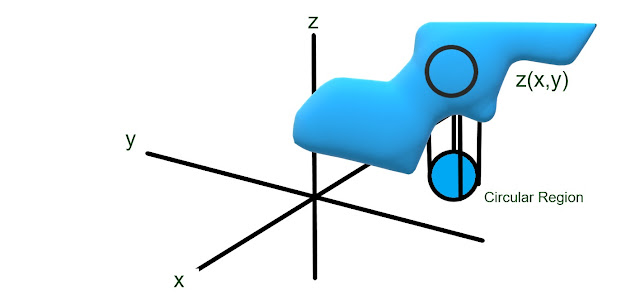## Thursday, December 13, 2018

### HP Prime and TI-84 Plus CE: Double Integral by Monte Carlo Method

HP Prime and TI-84 Plus CE:  Double Integral by Monte Carlo Method

Introduction

There are several ways to calculate integrals.  The most direct way is to find the anti derivative and evaluate it.  Another way is to use an approximation technique.   One technique to find a double integral is to evaluate random points on a given area (circle, rectangle, etc) and use the average in its calculations.

With the Monte Carlo method, we are at mercy of the random numbers selected.  Hence to improve our chances of getting a more accurate result, we have to use a large amount of random points for the calculation.  The flip side is that the Monte Carlo method, similar to the Romberg sum and Simpsons Method, finding the antiderivatve is not required.

The program MONTEINT calculates the double integral

∫ ∫ f(x,y) dx dy

over a circular area with center (X, Y) and radius R.   Please be sure the function is continuous and exists over the entire circular region.   In order to help with the calculation time is that the program runs the calculations several times and averages the results.  You enter the number of batches (B, number of times the calculation is made) and the number of sample points of each batch (N).A first for me:  this is the first diagram I drew and posted using Microsoft Paint 3D!

HP Prime Monte Carlo Double Integral Program: MONTEINT

EXPORT MONTEINT(f,C,D,R,B,N)
BEGIN
// 2018-12-11 EWS
// 'Z(X,Y)', center X,
// number of batches,
// batch size

LOCAL V,S,J,K,I;

V:=0;
FOR K FROM 1 TO B DO
S:=0;
J:=0;
FOR I FROM 1 TO N DO
X:=RANDOM(C-R,C+R);
Y:=RANDOM(D-R,D+R);
IF (X-C)^2+(Y-D)^2≤R^2 THEN

// for EVAL() to work,
// X and Y must be global
S:=S+EVAL(f);
J:=J+1;
END;
END;
V:=V+(π*R^2/J)*S;
END;
V:=V/B;
RETURN V;
END;

Note:  Enter f(X,Y) in single quotes.

TI 84 Plus Monte Carlo Double Integral Program: MONTEINT

Program MONTEINT

"2018-12-08 EWS"
Input "Z(X,Y) AS A STRING:",Y₀
FnOff 0
Disp "DISK"
Input "CENTER X: ",C
Input "CENTER Y: ",D
"SETUP VOLUME"
0→V
Input "BATCHES: ",B
Input "SAMPLE SIZE: ",N
"LOOPS"
For(K,1,B)
"SUM, TRUE COUNT"
0→S
0→J
For(I,1,N)
2*R*rand+(C-R)→X
2*R*rand+(D-R)→Y
If (X-C)²+(Y-D)²≤R²
Then
S+Y₀→S
J+1→J
End
End
V+(π*R²/J)*S→V
Disp "BATCH",K,"/",B
End
V/B→V
Disp "APPROX DOUBLE","INTEGRAL:",V

Note:  I put code that shows progress since the TI-84 Plus CE has a slower processor than the HP Prime.

Examples

Example 1:

f(X,Y) = √(LN(X + Y)) over a circle with center (5,5) of radius 1.   20 batches of 50 points each.

The value is approximately 4.76.

Example 2 - A Cautionary Tale:

f(X,Y) = X^2 + X*Y + Y^3 over a circle with center (0,0) of radius 2.  20 batches of 50 points each.

To illustrate how the Monte Carlo method is at the mercy of random points, the results vary between 11.3 to 13.1.

I am going to increase the number of batches to 30 with 100 points each.  Thankfully with the HP Prime's processing speed, this won't increase the calculation by a large amount.  The TI-84 Plus CE will require a longer wait.

After five trials, I get values between 12.07 and 13.15.

Increasing the number of points per batch to 200, I get results of 11.3 to 12.6.  The function f(X,Y) = X^2 + X*Y + Y^3  may not be a good function for the Monte Carlo method.

CAUTION:  The Monte Carlo is a method to calculate double integrals but it's only for approximate values only.  Several evaluations may be necessary to get a satisfactory approximation.

Eddie

Source:

Ward Cheney and David Kincaid.  Numerical Mathematics and Computing Sixth Edition Thomson Brooks/Cole: Belmont, CA 2008  ISBN-13 978-0-495-11475-8

All original content copyright, © 2011-2018.  Edward Shore.   Unauthorized use and/or unauthorized distribution for commercial purposes without express and written permission from the author is strictly prohibited.  This blog entry may be distributed for noncommercial purposes, provided that full credit is given to the author.  Please contact the author if you have questions.